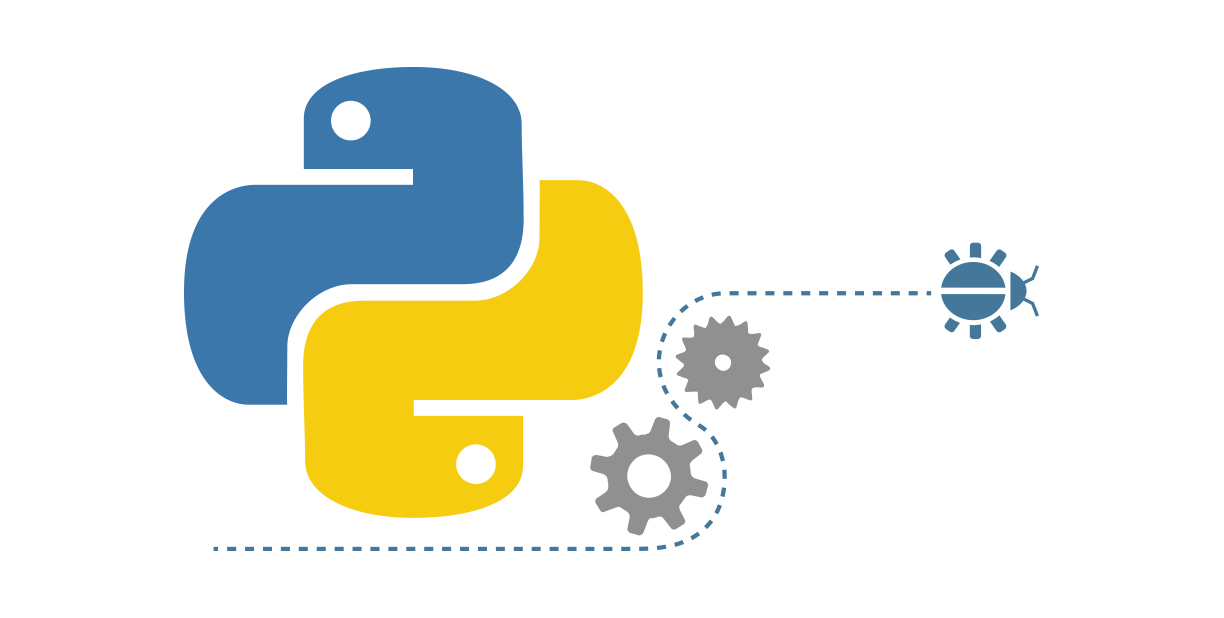``````import numpy
import matplotlib.pyplot as plt
import math
from keras.models import Sequential
from keras.layers import Dense
from keras.layers import LSTM
from sklearn.preprocessing import MinMaxScaler
from sklearn.metrics import mean_squared_error
%matplotlib inline``````

%matplotlib作用

1. 是在使用jupyter notebook 或者 jupyter qtconsole的时候，才会经常用到%matplotlib，也就是说那一份代码可能就是别人使用jupyter notebook 或者 jupyter qtconsole进行编辑的。关于jupyter notebook是什么，可以参考这个链接：Jupyter Notebook介绍、安装及使用教程
2. 而%matplotlib具体作用是当你调用matplotlib.pyplot的绘图函数plot()进行绘图的时候，或者生成一个figure画布的时候，可以直接在你的python console里面生成图像。

``````fig = plt.figure(facecolor='white')